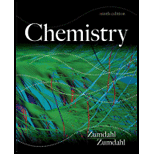# A certain reaction has the form aA → Products At a particular temperature, concentration versus time data were collected. A plot of 1/ [A] versus Lime (in seconds) gave a straight line with a slope of 6.90 × 10 −2 . What is the differential rate law for this reaction? What is the integrated rate law for this reaction? What is the value of the rate constant for this reaction? If [A] 0 for this reaction is 0.100 M, what is the first half-life (in seconds)? If the original concentration (at t = 0) is 0.100 M, what is the second half-life (in seconds)?### Chemistry

9th Edition
Steven S. Zumdahl
Publisher: Cengage Learning
ISBN: 9781133611097### Chemistry

9th Edition
Steven S. Zumdahl
Publisher: Cengage Learning
ISBN: 9781133611097

#### Solutions

Chapter
Section
Chapter 12, Problem 98CWP
Textbook Problem

## Expert Solution

### Want to see the full answer?

Check out a sample textbook solution.See solution

### Want to see this answer and more?

Experts are waiting 24/7 to provide step-by-step solutions in as fast as 30 minutes!*

See Solution

*Response times vary by subject and question complexity. Median response time is 34 minutes and may be longer for new subjects.